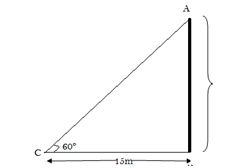# RRB ALP 2018 Practice Test Papers | Arithmetic Questions (Day-59)

Dear Aspirants, Here we have given the Important RRB ALP & Technicians Exam 2018 Practice Test Papers. Candidates those who are preparing for RRB ALP 2018 can practice these Arithmetic Questions to get more confidence to Crack RRB 2018 Examination.

[WpProQuiz 2798]

Click “Start Quiz” to attend these Questions and view Solutions

1. Divide 8√15 by 2√3
1. 2√3
2. 4√5
3. 8√3
4. 3√2
1. The degree of a non-zero constant polynomial is
1. One
2. Two
3. Zero
4. Infinite
1. A box contains 3 blue, 2 white, and 4 red marbles. If a marble is drawn at random from the box, what is the probability that it will be white?
1. 5/9
2. 2/ 9
3. 1 /9
4. 4 / 9
1. A tower stands vertically on the ground. From a point on the ground, which is 15 m away from the foot of the tower, the angle of elevation of the top of the tower is found to be 60°. Find the height of the tower.
1. 15√3
2. 5√3
3. 3√5
4. 15
1. The circumference of circle is equal to perimeter of a square. If side of the square is 11cm. Find the Area of that Circle?
1. 121 cm^2
2. 132 cm^2
3. 154 cm^2
4. 616 cm^2
1. A fridge is marked up that selling price above 20 % on its cost price. After selling with a discount a shop keeper makes a profit of 8%. What is the cost price of the fridge, if the difference between the cost price and selling price of the fridge is 360?
1. 1200
2. 3000
3. 5400
4. 2000
1. Simplify

19×53 × 25 ÷ 125= ?

1. 203.4
2. 202.4
3. 200.4
4. 201.4
1. When a number is added with its thrice value becomes 60. Find the number?
1. 15
2. 10
3. 12
4. 8
1. Average age of 30 students is 24. When a teacher added with the students the average increased by 1. Find the age of that teacher.
1. 25
2. 55
3. 45
4. 30
1. Find the value of x

10% of 30% of 50% of x = 45÷5

1. 300
2. 600
3. 1200
4. 900

8√15 ÷ 2√3 = 8√3 ×√5 ÷ 2√3 =  4√5

The degree of a non-zero constant polynomial is zero.

The number of outcomes favorable to the event W = 2

So, P(W) =2/9

AB/BC =tan 60

√3=  AB/ 15

AB = 15√3Side = 11 cm ==> Perimeter of a Square = 4× 11 = 44 cm

Area of square = Perimeter of Circle

2Πr = 44

R = 7

Πr^2 = 22/7 × 7×7 = 154

Let take c.p = 100

M.P = 120

S.P = 108

120 – 108 = 12

12 ———— 360

100 ———— ?

(360× 100)/ 12 = 3000

X + 3x = 60

4X = 60

X = 15

X + 3x = 60

4X = 60

X = 15

Total age of students = 30×24 = 720

Total age of student and teacher = 31× 25 = 775

Age of the teacher is = 775 – 720 = 55